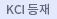#검색
다국어 입력

http://chineseinput.net/에서 pinyin(병음)방식으로 중국어를 변환할 수 있습니다.

변환된 중국어를 복사하여 사용하시면 됩니다.

예시)
• 中文 을 입력하시려면 zhongwen을 입력하시고 space를누르시면됩니다.
• 北京 을 입력하시려면 beijing을 입력하시고 space를 누르시면 됩니다.
닫기

검색결과 좁혀 보기

• 좁혀본 항목 보기순서

• 원문유무
• 음성지원유무
• 원문제공처
• 등재정보
• 학술지명
• 주제분류
• 발행연도
• 작성언어
• 저자

오늘 본 자료

• 오늘 본 자료가 없습니다.
더보기
• 무료
• 기관 내 무료
• 유료
•상사해법을 이용한 쐐기형 물체 주위의 미세 극성유체 유동 특성에 관한 연구

A similarity solution of a steady laminar flow of micropolar fluids past wedges has been studied. The similarity variables found by Falkner and Skan are employed to reduce the streamwise-dependence in the coupled nonlinear boundary layer equations. Numerical solutions of the equations are then obtained using the fourth-order Runge-Kutta method and the distribution of velocity, micro-rotation, shear and couple stress across the boundary layer are obtained. These results are compared with the corresponding flow problems for Newtonian fluid past wedges with various wedge angles. Numerical results show that, keeping ${\beta}$ constant, the skin friction coefficient is lower for a micropolar fluid, as compared to a Newtonian fluid. For the case of constant material parameter K, however, the velocity distribution for a micropolar fluid is higher than that of a Newtonian fluid.

• 압력 평형식 온도조절 밸브 내부 유동 특성에 대한 수치적 연구

Temperature Control Valve (TCV) is one of the useful temperature control devices, which is used to control constant temperature of working fluid in power and chemical plants and domestic water supply systems. TCV is composed of body, cylinder and piston, and the body shape has a symmetrical H-type. In general, it has several inlet and outlet holes, and its shape is like as tubular sleeve. The piston has three rings: two rings of the end of piston have the function of controlling inlet flow rate with hot and cold working fluids, the center ring has the function of preventing hot and cold water from intermixing. Consequently, the shapes of piston and cylinder are the main design parameters in the performance of TCV. In this study, numerical analyses were carried out with two different piston and cylinder shapes to investigate the functions as a temperature control valve and the flow characteristics according to piston opening grade in TCV. Using a commercial code, FLUENT, velocity and pressure fields in TCV are obtained under steady, standard k-ε turbulence model and no-slip condition.

• 양흡입 펌프 내부 유동특성에 관한 수치적 연구

The flow characteristics of double suction pump are investigated by numerically. Calculations are performed by using SIMPLE algorithm at the design and off-design points. Symmetric nature of flow fields in blade channels is discovered at design point, but asymmetirc effects are discovered at the off-design point. Numerical results show that the formation of secondary flow in volute of double suction pump shows different trends when compared with the case of single suction pump. Also results show that double vortices are formed in the volute cross section.

• CVD 반응기 내에서의 유동장에 대한 샤워헤드 지름의 영향에 대한 수치적 연구

Plasma Enhanced Chemical Vapor Deposition (PECVD) process uses unique property of plasma to modify surfaces and to achieve the high deposition rates. In this study, a vertical thermal RF-PECVD (Radio Frequency-PECVD) reactor is modeled to investigate thermal flow and the deposition rates with various shapes of the showerhead. The showerhead in the CVD reactor has the shape of a ring and gases are injected in parallel with the susceptor, which is a rotating disk. In order to achieve the high deposition rates, we have simulated the thermal flow fields in the reactor with several showerhead models. Especially the effects of the number of injection holes and the rotating speed of the susceptor are studied. Using a commercial code, CFDACE, which uses FVM (Finite Volume Method) and SIMPLE algorithm, governing equations have been solved for the pressure, mass-flow rates and temperature distributions in the CVD reactor. With the help of the Nusselt number and Sherwood number, the heat and mass transfers on the susceptor are investigated. In order to characteristics of measure the flatness of the layer, furthermore, the relative growth rate (RGR) is considered.

• 냉각홀 형상 변화에 따른 원형봉 선단의 막냉각 특성 연구

Dispersion of coolant jets in a film cooling flow field is the result of a highly complex interaction between the film cooling jets and the mainstream. In order to investigate the effect of blowing ratios on the film cooling of turbine blade, cylindrical body model was used. Mainstream Reynolds number based on the cylinder diameter was 7.1×10⁴, The effect of coolant flow rates was studied for blowing ratios of 0.7, 0.9, 1.2 and 1.5, respectively. The temperature distribution of the cylindrical model surface is visualized by infrared thermography (IRT). Results show that the film-cooling performance could be significantly improved by the shaped injection holes. For higher blowing ratio, the spanwise-diffused injection holes are better due to the lower momentum flux away from the wall plane at the hole exit.